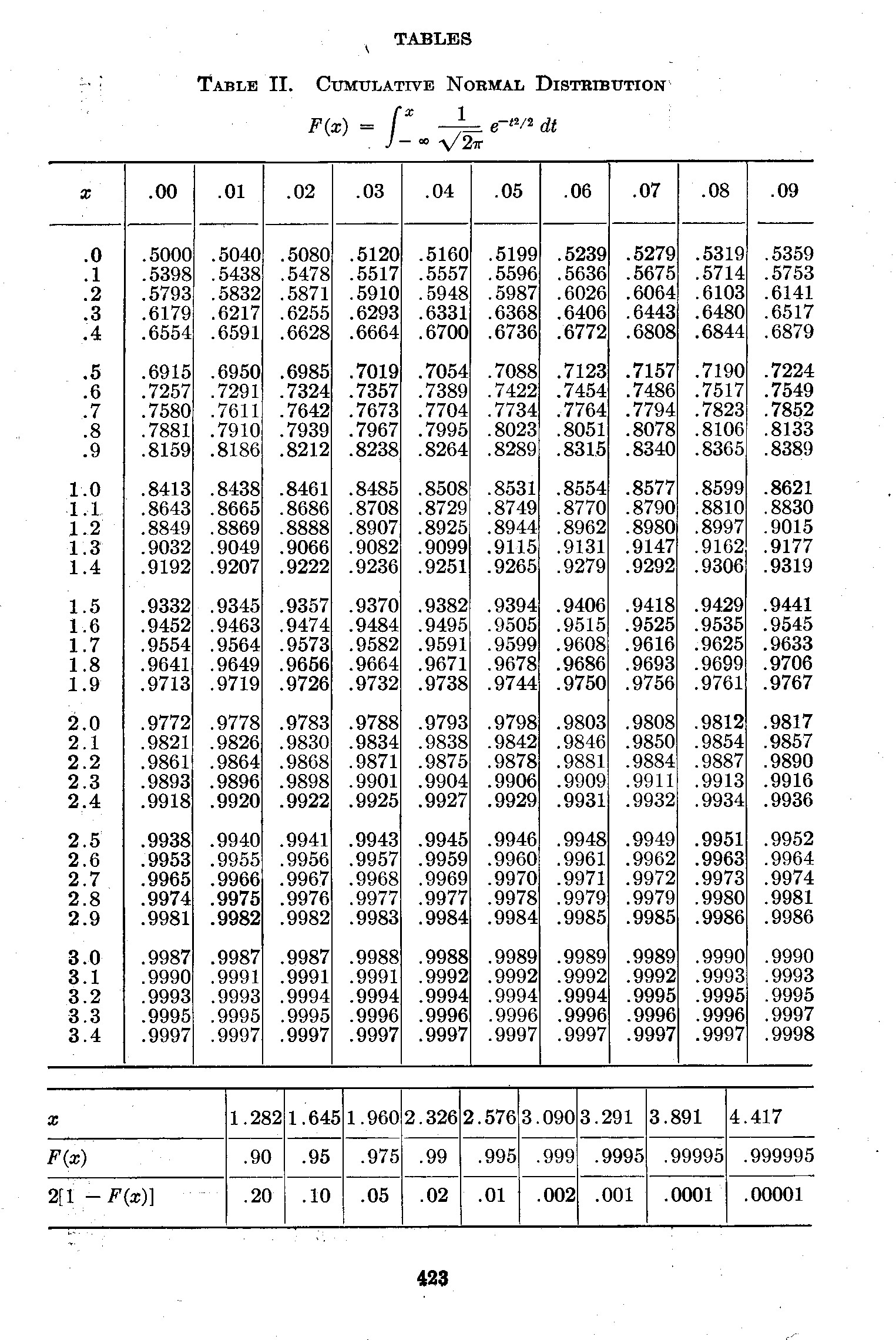## FOUR INDEPENDENT MEASUREMENTS OF VULCAN’S PERIOD

© Copyright: 19 Mar. 2014 updated 29 Jul. 2015, 7 Aug. 2016
PREVIOUS COMBINED PERIODS

This Vulcan web site presents a way to compute Vulcan’s orbital parameters by using an Blavetsky's theosophy and an IRAS (Infrared Astronomical Survey) object cataloged as 1732+239. This results in a theoretical value for Vulcan period to be 4969.0 +/- 5.75 (one sigma) years.

However, there are three other methods that lead directly to four independent computations of Vulcan’s orbital period. The first was preformed by Professor Forbes in 1880 and used the aphelion’s of long period comets. The second was the reconstruction of the path of a giant comet, CR105 over a period of that estimated to be two Vulcan’s period and measuring the time between three successive positions on the comet’s orbit. This comet has been shown to be one of the ones in a 3:2 resonance with Vulcan’s orbital period and the total of three consecutive periods must exactly match that of two of Vulcan’s periods. The third way involves measuring the time interval between weather changes believed to be cause by comet impacts (or possibly by their simply exploding in the atmosphere). The specific clusters of comets within two comet swarms were used in this calculation, Swarm A: Cl-1 and Swarm B:Cl-2. Where only the average comet period and its one sigma error was obtained, these values were multiplied by 3/2 to translated them back into the values reflecting the period of Vulcan. These are listed below.

• Forbes 5000 years +/- 200 (one sigma) years
• CR105 [3319.3 years +/- 19.5 (one sigma) years] x 3/2 = 4979 years +/- 29.25 (one sigma) years
• Swarm A: Cl-1 4969.5 years +/- 10 (one sigma) years
• Swarm B:Cl-1d 4971.0 years +/- 16 years

The combined one sigma error is obtained by adding the inverse sum of the squares and taking the square root. Thus:

1/(one combined sigma)2 = 1/2002 + 1/29.252 + 1/102 + 1/162 = 0.01510007 . . . EQ A

Thus, one combined sigma = 8.14 years

The Combined Period is obtained by adding the sums of the separate periods, each divided by its separate one sigma value, Then dividing this quantity by 1/(one combined sigma)2. Thus:

(5000/2002 + 4979/29.252 + 4969.5/102 + 4971.0/162)/0.01510007 = 75.057528/0.01510007 = 4970.70 . . . EQ B

Calculation corrected due to refinement of CR105 orbital period changing from our 3316.7 years (unaffected by Vulcan and its orbit) to 3305 years. The difference in the (no Vulcan) orbit 3316.7 to 3319.3 years (affected by Vulcan and its orbit) is assumed to remain the same, 2.6 years. Since CR105's orbital period is actually 3305 + 2.6 = 3307.6 years. Since CR105 is a detached object not influenced by Neptune, it is a pure 3:2 resonate orbit with Vulcan. Thus, Vulcan's orbit from this new CR105 data is 3307.6 X (3/2) = 4961.4 years.

The combined calculations for Vulcan's period equation above (EQ B) can be modified by adding this time term
X = (4961.4/29.252 - 4979/29.252)/0.01510007
or
X = (-17.6/29.252)/0.01510007 = -0.02057/0.01510007 = -1.36227
to make this minor correction. Thus;
4970.70 - 1.36227 = 4969.338 years

Then, Vulcan's Combined Period from these measurements is = 4969.3 years +/- 8.14 (one sigma) years . . . EQ C

A statistical estimate can be made to determine how well the combined period data compares to the theoretical value for Vulcan period to the Combined Period value. This method measures how far they are apart from a Gaussian perspective and that can be translated into a probability of overlap.Figure: Gaussian Separation VS. Probability of Association

Gaussian Separation = [Absolute Value of the (Theoretical Period - Combined Period)]/[( one sigma)2 + (one combined sigma)2]1/2

Gaussian Separation = [Absolute Value(4969 - 4969.3)/[(5.752 + 8.142)]1/2

Gaussian Separation = 0.3/9.964 = 0.030

This translates into a 97.6% chance that the combined measured values (4970.70 years) is related to the theoretical value (4969 years).

A similar calculation can be made for the case where Vulcan is not constrained to be at the IRAS point. Here the theoretical estimate is 4972 Years +/- 27.35

Gaussian Separation = [Absolute Value(4972 - 4969.3)/( 27.352 + 8.142)] = 2.7/[814.2821]1/2 = 2.7/28.535 = 0.0946

In this case, the Gaussian Separation = 0.0946 which corresponds to a 92.4% chance that the theoretical and combined values are associated. Thus this statistical analysis cannot resolve whether or not Vulcan is exactly at the specified IRAS point, or is slightly removed from it (as if a typo in recording the data was made).